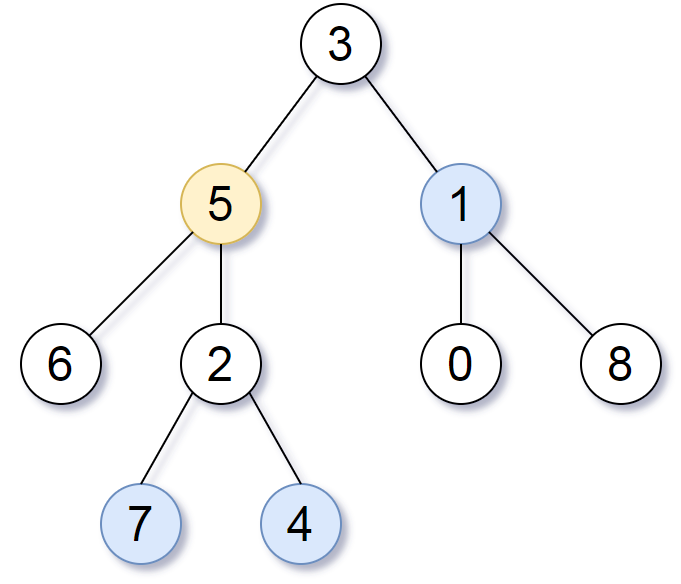Formatted question description: https://leetcode.ca/all/863.html

# 863. All Nodes Distance K in Binary Tree (Medium)

We are given a binary tree (with root node root), a target node, and an integer value K.

Return a list of the values of all nodes that have a distance K from the target node.  The answer can be returned in any order.

Example 1:

Input: root = [3,5,1,6,2,0,8,null,null,7,4], target = 5, K = 2

Output: [7,4,1]

Explanation:
The nodes that are a distance 2 from the target node (with value 5)
have values 7, 4, and 1.Note that the inputs "root" and "target" are actually TreeNodes.
The descriptions of the inputs above are just serializations of these objects.


Note:

1. The given tree is non-empty.
2. Each node in the tree has unique values 0 <= node.val <= 500.
3. The target node is a node in the tree.
4. 0 <= K <= 1000.

Companies:

Related Topics:

## Solution 1. DFS + Level Order

Consider a simpler question: given root, find the nodes that have a distance K from the root node. We can simply use level order traversal to find the nodes at Kth level.

For this question, we need to consider the target’s parents and the nodes in parents’ substrees. We can use DFS to locate the target node, and if target node is found, return the distance to target node; otherwise return INT_MAX which means the current node is not target’s parent and should be ignored.

For the target’s parents, we use the level order traversal in the other subtree that doesn’t contain target.

// OJ: https://leetcode.com/problems/all-nodes-distance-k-in-binary-tree/

// Time: O(N)
// Space: O(N)
class Solution {
private:
vector<int> ans;
TreeNode *target;
int K;
int inorder(TreeNode *root) {
if (!root) return INT_MAX;
if (root == target) return 1;
int left = inorder(root->left);
if (left != INT_MAX) {
if (left < K) levelOrder(root->right, K - left - 1);
else if (left == K) ans.push_back(root->val);
return left + 1;
}
int right = inorder(root->right);
if (right != INT_MAX) {
if (right < K) {
levelOrder(root->left, K - right - 1);
} else if (right == K) ans.push_back(root->val);
return right + 1;
}
return INT_MAX;
}
void levelOrder(TreeNode *root, int K) {
if (!root) return;
queue<TreeNode*> q;
q.push(root);
int level = 0;
while (q.size()) {
int cnt = q.size();
while (cnt--) {
TreeNode *node = q.front();
q.pop();
if (level == K) ans.push_back(node->val);
else {
if (node->left) q.push(node->left);
if (node->right) q.push(node->right);
}
}
++level;
}
}
public:
vector<int> distanceK(TreeNode* root, TreeNode* target, int K) {
this->target = target;
this->K = K;
levelOrder(target, K);
inorder(root);
return ans;
}
};


## Solution 2. BFS

Keep track of the mapping between node and its parent. BFS from target node.

// OJ: https://leetcode.com/problems/all-nodes-distance-k-in-binary-tree/

// Time: O(N)
// Space: O(N)
class Solution {
private:
unordered_map<TreeNode*, TreeNode*> parent;
void dfs(TreeNode *node, TreeNode *par) {
if (!node) return;
parent[node] = par;
if (node->left) dfs(node->left, node);
if (node->right) dfs(node->right, node);
}
public:
vector<int> distanceK(TreeNode* root, TreeNode* target, int K) {
dfs(root, NULL);
unordered_set<TreeNode*> seen;
seen.insert(NULL);
queue<TreeNode*> q;
vector<int> ans;
q.push(target);
while (q.size()) {
int cnt = q.size();
while (cnt--) {
root = q.front();
seen.insert(root);
q.pop();
if (!K) ans.push_back(root->val);
else {
if (seen.find(root->left) == seen.end()) q.push(root->left);
if (seen.find(root->right) == seen.end()) q.push(root->right);
if (seen.find(parent[root]) == seen.end()) q.push(parent[root]);
}
}
--K;
}
return ans;
}
};


Java

/**
* Definition for a binary tree node.
* public class TreeNode {
*     int val;
*     TreeNode left;
*     TreeNode right;
*     TreeNode(int x) { val = x; }
* }
*/
class Solution {
public List<Integer> distanceK(TreeNode root, TreeNode target, int K) {
Map<TreeNode, String> nodePathMap = new HashMap<TreeNode, String>();
nodePathMap.put(root, "");
queue.offer(root);
while (!queue.isEmpty()) {
TreeNode node = queue.poll();
String path = nodePathMap.getOrDefault(node, "");
TreeNode left = node.left, right = node.right;
if (left != null) {
nodePathMap.put(left, path + "0");
queue.offer(left);
}
if (right != null) {
nodePathMap.put(right, path + "1");
queue.offer(right);
}
}
String targetPath = nodePathMap.getOrDefault(target, "");
List<Integer> valuesList = new ArrayList<Integer>();
Set<TreeNode> set = nodePathMap.keySet();
for (TreeNode node : set) {
String path = nodePathMap.getOrDefault(node, "");
if (distance(targetPath, path) == K)
}
return valuesList;
}

public int distance(String path1, String path2) {
int length1 = path1.length(), length2 = path2.length();
int minLength = Math.min(length1, length2);
int startIndex = 0;
while (startIndex < minLength) {
char c1 = path1.charAt(startIndex), c2 = path2.charAt(startIndex);
if (c1 != c2)
break;
startIndex++;
}
int distance = length1 - startIndex + length2 - startIndex;
return distance;
}
}